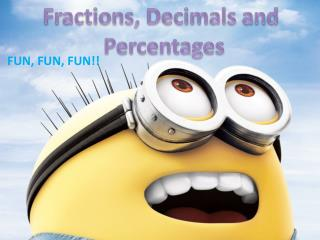DownloadDownload PresentationFUN, FUN, FUN!!

# FUN, FUN, FUN!!

Télécharger la présentation## FUN, FUN, FUN!!

- - - - - - - - - - - - - - - - - - - - - - - - - - - E N D - - - - - - - - - - - - - - - - - - - - - - - - - - -
##### Presentation Transcript

1. Fractions, Decimals and Percentages FUN, FUN, FUN!!

2. Those three mathematic terms are all related. They are all out of a whole. 0.20. That is how decimals are shown e.g 0.65, 0.98, 0.50 and so on. Fractions Decimals and Percentages 20% that is how percentages are shown e.g 65%, 98% 50% and so on. (Percentages are basically fractions out of 100) 50 That is how fractions are shown e.g 100 65 98 50 100 100 100 Fractions are parts of a whole and can be out of any numbers

3. Game Link! Fractions http://www.sheppardsoftware.com/mathgames/fractions/memory_equivalent1.htm Fractions are parts of a whole and are represented like this: ½ ¾ The number on the bottom is called ‘Denominator’. It tells you how many parts a whole is divided into. Fractions are also used for discount sales. ½ price means that the price is now half the normal one. The number on the top is called ‘Numerator’. It tells you how many parts you have from the denominator. ¾ means 3 parts took from something divided into 4. 2 Numerator ½ 3 Denominator

4. Decimals Game Link! http://www.sheppardsoftware.com/mathgames/fractions/FractionsToDecimals.htm Easy as pie Decimals are similar to fractions. However, they can only be out of numbers divisible by ten. They are represented like this. 1.11. The numbers on the right side of the decimal point are numbers smaller than one. 1.1 Decimal Point

5. Percentages Game Link! http://www.kidsmathgamesonline.com/numbers/percentages.html Percentages are fractions out of 100. However, they are shown differently. This is how percentages are written: 50%, 40%. Percentages are mainly used in discount sales. If it’s 50% off, it means if the sale item was \$100, the price will be \$50 less.

6. for listening